# 1. We reject the null hypothesis only when: a. our sample mean is larger than the population mean. b. the p value asso...

1. We reject the null hypothesis only when:
a. our sample mean is larger than the population mean.
b. the p value associated with our test statistic is greater than the significance level of the test
we have chosen.
c. our sample mean is smaller than the population mean.
d. the p value associated with our test statistic is smaller than the significance level of the test
we have chosen.

2. In a study of simulated juror decision making, researchers investigated whether there was a
relationship between the type of verdict and the type of abuse experienced by the defendant. Half
of the participants read a 12 page summary of a case where, a physically abused woman, shot
and killed her husband, while the other half of the participants read a 12 page summary of a case
where, an emotionally abused woman, shot and killed her husband. Participants were then asked
to assign a verdict (not guilty or guilty). After analysis, the researchers failed to reject the null
hypothesis. What conclusions can they make?
a. There are more not guilty verdicts, when there is physical abuse.
b. There are more not guilty verdicts, when there is emotional abuse.
c. There is a no relationship between verdicts and type of abuse.
d. There is a relationship between verdicts and type of abuse.

3. The p value is the:
a. probability with which a test statistic would occur if the research hypothesis were true.
b. probability with which a test statistic would occur if the null hypothesis were true.
c. cutoff probability at which a test statistic is considered extreme.
d. probability with which a test statistic would occur if both the null and research hypothesis
were false.

Please use the following information to answer questions 4, 5, 6, and 7:
Researchers conducted a study to assess the notion that women talk more than men by
comparing the number of words uttered, per day, by each sex. Volunteer participants wore
inconspicuous recording devices that recorded their daily word usage. Based on the study
results (contained in the following table), is there any validity to the notion that women talk
more than men do?

Number of Participants Mean Standard Deviation
Women 5 16,200.80 1779.45
Men 5 15,989.40 2229.85

4. What is the appropriate hypothesis test?
a. z-test
b. t-test for matched pairs
c. t-test for independent samples
d. chi square test for independence

5. What are the null and alternative hypotheses?
a. Ho:?1=?2; Ha:?1<?2
b. Ho:?1??2=0; Ha:?1??2?0
c. Ho:?1??2=0; Ha:?1??2<0
d. Ho:?1??2=0; Ha:?1??2>0

6. What is the value of the test statistic?
a. .166
b. .264
c. .118
d. 7.46

7. The p-value obtained for this study was .8727. What do these results tell us?
a. the data provide sufficient evidence to reject H0; therefore, we conclude that there are no
differences in number of words uttered daily by women and the number of words uttered
daily by men.
b. the data provide sufficient evidence to reject H0; therefore, we conclude that the number
of words uttered daily by women is greater than the number of words uttered daily by
men.
c. the data do not provide sufficient evidence to reject H0; therefore, we conclude that there
are no difference in number of words uttered daily by women and the number of words
uttered daily by men.
d. the data do not provide sufficient evidence to reject H0; therefore, we conclude that the
number of words uttered daily by women is greater than the number of words uttered daily
by men.

Please use the following information to answer questions 8, 9, 10, 11, and 12:
It is known that the population mean for the verbal section of the SAT is 500, with a standard
deviation of 100. In 2006, a sample of 100 students taking the SAT, whose family income was less
than $10,000, had a verbal SAT score of 429. Perform a one-tailed hypothesis test to determine whether the group, whose family income was less than$10,000, scored significantly lower on
average than the population.
8. What is the appropriate hypothesis test?
a. t-test for matched pairs
b. z-test
c. t-test for independent samples
d. chi square test for independence

9. What are the null and alternative hypotheses?
a. H0:?=?0; Ha:?<?0
b. H0:?=?0; Ha:?>?0
c. H0:?=?0; Ha:???0
d. None of these are correct

10. What is the value of the test statistic?
a. -.71
b. .71
c. -7.1
d. +7.1
11. What is the p-value for the test statistic (based on rounded values)?
a. .2389
b. .7611
c. 1.000
d. .0000

12. Using the significance level of .05, do the results lead to rejection of the null hypothesis?
Please describe what the results tell us about mean verbal SAT scores for those with a
family income is less than $10,000. a. Yes, reject the null hypothesis. The mean Verbal SAT score for students, whose family income was less than$10,000, is not significantly lower than then the average Verbal SAT
score for the population.
b. Yes, reject the null hypothesis. The mean Verbal SAT score for students, whose family
income was less that $10,000, is significantly lower than then the average Verbal SAT score for the population. c. No, do not reject the null hypothesis. The mean Verbal SAT score for students, whose family income was less than$10,000, is not significantly lower than then the average
Verbal SAT score for the population.
d. No, do not reject the null hypothesis. The mean Verbal SAT score for students, whose
family income was less than $10,000, is significantly lower than then the average Verbal SAT score for the population. 13. Researchers investigated the effects of encoding conditions on subsequent memory for a list of words. Participants were assigned to one of three encoding conditions: 1) saw list of nouns they were asked to remember (word alone condition), 2) list of words was accompanied by a picture (word + picture condition), or 3) list of words was accompanied by sound effects matching the object (sound-effects condition). A week later, researchers measured the proportion of words that participants got correct on a word recognition test. The researchers analyzed the data using an ANOVA, which resulted in the following table: Analysis of Variance for Encoding Conditions DF SS MS F P Group 2 .177 .089 13.129 0.000 Error 9 .061 .007 Total 11 .238 Using the above table, we can conclude that: a. the data provide strong evidence that subsequent memory for words are related to encoding conditions. b. the data provide strong evidence that subsequent memory for words are related to encoding conditions in the following way: The proportion of words remembered by people who are in the sound-effects condition is higher than the proportion of words remembered by people who are in the word + picture condition, which in turn is higher than the proportion of words remembered by people who are in word alone condition. c. the data provide strong evidence that the three word proportion scores (representing the three encoding conditions) are all equal. d. the data do not provide sufficient evidence that subsequent memory for words is related to encoding conditions. 14. A researcher, who was interested in gender differences, conducted a study to determine whether there is a difference between the weekly allowances given to 10 year old boys and the weekly allowances given to 10 year old girls. The researcher asked thirty 10 year old boys and thirty 10 year old girls, the amount they received for their weekly allowance. What would be the appropriate hypothesis testing technique to analyze the data from this study? a. t-test for two independent samples b. t-test for matched pairs c. z-test for population mean d. correlation 15. Valium is a drug that is used in the treatment of anxiety. A researcher was interested in determining the effect of Valium on the motor performance of rats. Thirty rats were randomly assigned to one of the following three groups: 1) low dose of Valium, 2) high dose of Valium, or 3) placebo (saline water). After injection with the assigned substance, the rats were immediately placed in a Skinner box and the number of bar presses made in 5 minutes was recorded by the researcher. What hypothesis testing technique should the researcher use to analyze the data from this study? a. t-test for two independent samples b. z-test for population mean c. Chi square test for independence d. ANOVA 16. A psychologist, who studies sleep and memory, is interested in determining whether shortterm memory is affected by sleep loss. A group of participants is administered a shortterm memory test at 9 am on Day 1 and again at 9 am on Day 2, after the participants were kept awake all night. What hypothesis testing technique should the researcher use to determine whether sleep loss affects shortterm memory? a. t-test for two independent samples b. Chi square test for independence c. t-test for matched pairs d. correlation 17. A light bulb manufacturer advertises that its 75 watt bulbs have an average life of 1000 hours. A consumer product advocacy group wanted to determine whether or not this is false advertising. The advocacy group bought 30 bulbs and burned them until they failed. They found that the light bulbs burned an average of 1217 hours with a standard deviation of 216.93 hours. What hypothesis testing technique should be used to determine whether or not the average life time of the sample of light bulbs is different from the advertised life time? a. t-test for population mean b. z-test for population mean c. t-test for two independent samples d. t-test for matched pairs 18. A resident of Hoboken, New Jersey, wondered whether the average cost of a parking ticket in Hoboken, differed from that in New Jersey cities as a whole. Public records from city parking utilities across the state revealed that in 2007, the average cost of a parking ticket in the state was$45, with a standard deviation of $11. In that same year, the average cost of a sample of 15 parking tickets in Hoboken totaled$57. What hypothesis test should the resident use to
analyze the data?
a. t-test for two independent samples
b. z-test for population mean
c. t-test for matched pairs
d. t-test for population mean

19. A professor is interested in determining whether assigning mandatory homework affects
students

Concepts and reason

Statistical hypotheses testing: Hypotheses testing is used to make inferences about the population based on the sample data. The hypotheses test consists of null hypothesis and alternative hypothesis.

Null hypothesis: The null hypothesis states that there is no difference in the test, which is denoted by ${H_0}$ . Moreover, the sign of null hypothesis is equal $\left( = \right)$ , greater than or equal $\left( \ge \right)$ and less than or equal $\left( \le \right)$ .

Alternative hypothesis: The hypothesis that differs from the ${H_0}$ is called alternative hypothesis. This signifies that there is a significant difference in the test. The sign of alternative hypothesis is less than $\left( < \right)$ , greater than $\left( > \right)$ , or not equal $\left( \ne \right)$ .

P-value method approach: If the probability value less than or equal to the level of the significance, then reject the null hypothesis. Moreover, the probability of obtaining the value from the sample test statistic which is extremely small that can be found in the sample data by assumption of null hypothesis is being true.

Fundamentals

Rejection rule based on p-value:

If $p{\rm{ - value}} \le \alpha$ then reject null hypothesis.

Rejection rule for critical value method:

If ${\rm{Test statistic}} \ge {z_\alpha }$ , then reject the null hypothesis ${H_0}$ .

(1)

The rejection rule states that, the null hypothesis is rejected if p-value is less than the level of significance and the null hypothesis is not rejected if the p-value is greater than the level of significance.

(2)

The experiment is conducted to test whether there is a relationship between the type of verdict and the type of abuse experienced by the defendant.

State the hypotheses.

Null hypothesis:

${H_0}:$ There is no relationship between the type of verdict and the type of abuse.

Alternative hypothesis:

${H_a}:$ There is relationship between the type of verdict and the type of abuse.

From that the given information, the researcher failed to reject the null hypothesis. This implies that there is no relationship between the type of verdict and the type of abuse.

(3)

The probability of getting the value of the statistic that is as extreme as the observed statistic considering the null hypothesis is true is called as p-value.

Ans: Part 1

The p-value associated with out test statistic is smaller than the significance level of the test we have chosen.

#### Earn Coin

Coins can be redeemed for fabulous gifts.

Similar Homework Help Questions
• ### Identify the null hypothesis, alternative hypothesis, test statistic, P-value, conclusion about the null hypothesis, and final...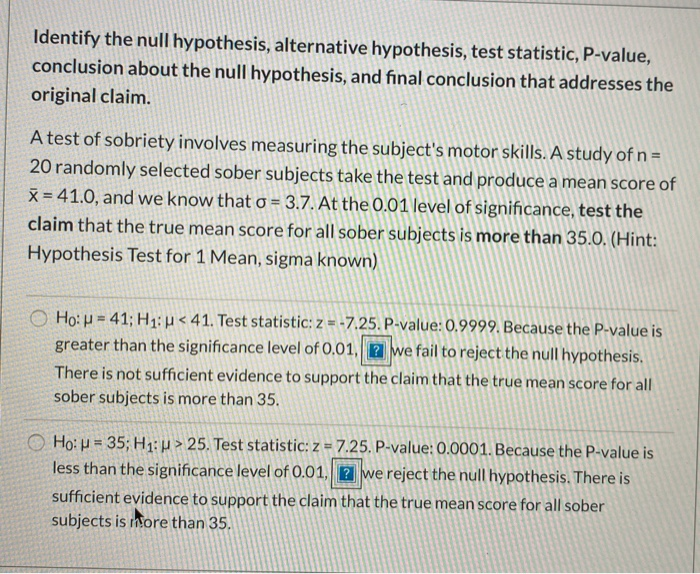Identify the null hypothesis, alternative hypothesis, test statistic, P-value, conclusion about the null hypothesis, and final conclusion that addresses the original claim. A test of sobriety involves measuring the subject's motor skills. A study of n = 20 randomly selected sober subjects take the test and produce a mean score of X = 41.0, and we know that o = 3.7. At the 0.01 level of significance, test the claim that the true mean score for all sober subjects is...

• ### A random sample from normal population yielded sample mean=40.8 and sample standard deviation= 6.1, n =...

A random sample from normal population yielded sample mean=40.8 and sample standard deviation= 6.1, n = 15. H0: μ = 32.6, Ha: μ ≠ 32.6, α = 0.05. Perform the hypothesis test and draw your conclusion. A. Test statistic: t = 5.21. P-value=0.00013. Reject H0. There is sufficient evidence to support the claim that the mean is different from 32.6. B. Test statistic: t = 5.21. P-value=1.9E-7 (0.00000019). Reject H0. There is sufficient evidence to support the claim that the...

• ### Options for "The P-value is" Greater than. Less than....... so reject or fail to reject...... There...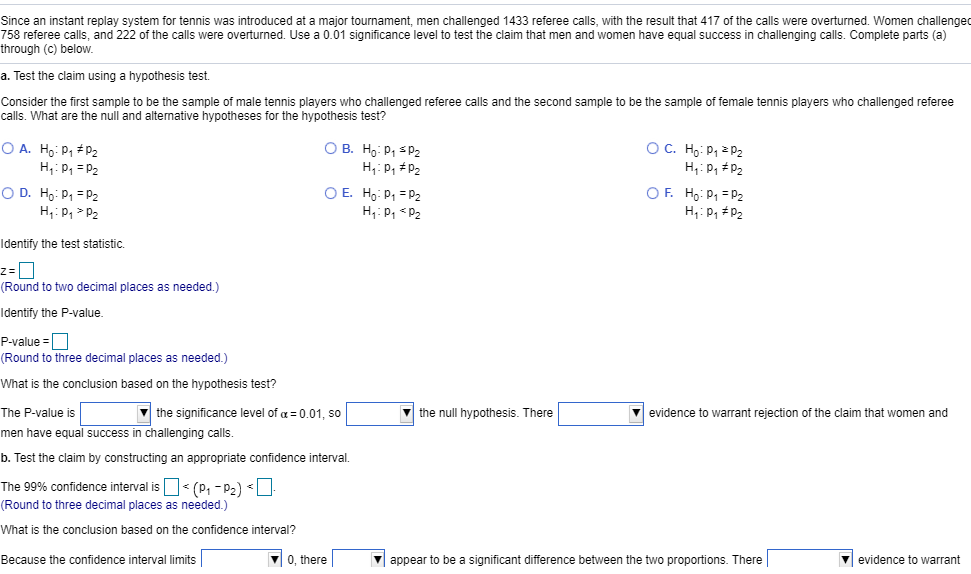Options for "The P-value is" Greater than. Less than....... so reject or fail to reject...... There is sufficient or nonsufficient evidence he Because the confidence interval limit include do not include does does not appear to be a significant difference between the two proportions. There is not sufficient is sufficient evidence to warrant rejection of the claim that men and Since an instant replay system for tennis was introduced at a major tournament, men challenged 1433 referee calls, with the...

• ### A. B. 2. The test​ statistic,is ______ ​(Round to two decimal places as​ needed.) 3. The​ P-value is ______ ​(Round t...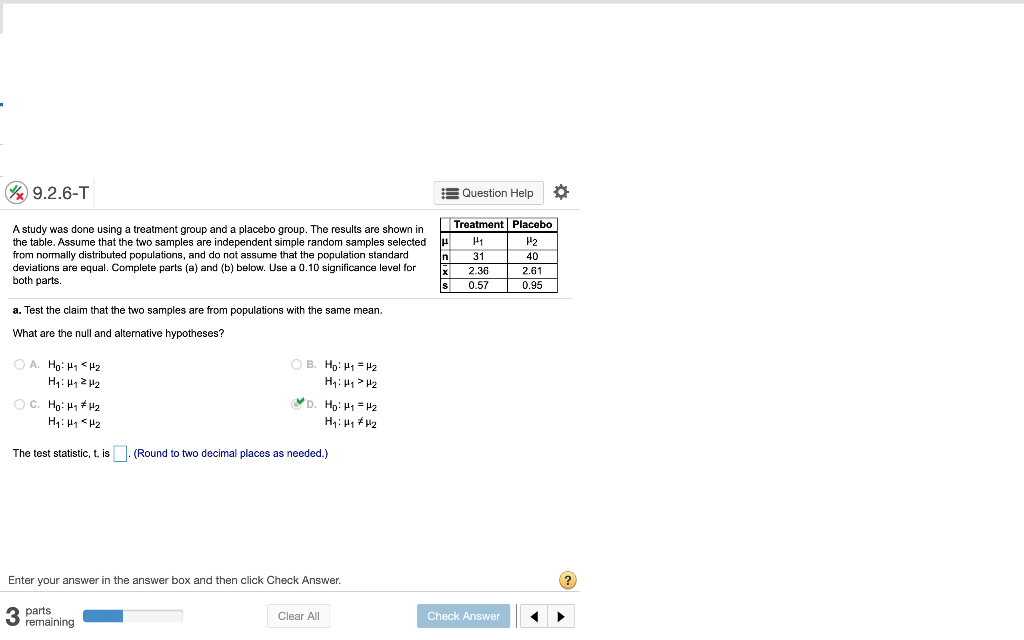A. B. 2. The test​ statistic,is ______ ​(Round to two decimal places as​ needed.) 3. The​ P-value is ______ ​(Round to three decimal places as​ needed.) 4. State the conclusion for the test. ________________ the null hypothesis. There ______ sufficient evidence to support the claim that those treated with magnets have a greater mean reduction in pain than those given a sham treatment. 5. Is it valid to argue that magnets might appear to be effective if the sample sizes...

• ### r joint null hypothesis test statistic comes . If ou out to be greater than the relevant critical value, do we reject or fail to reject the joint null hypothesis? S&W Chapter 9 -Assessing Stu...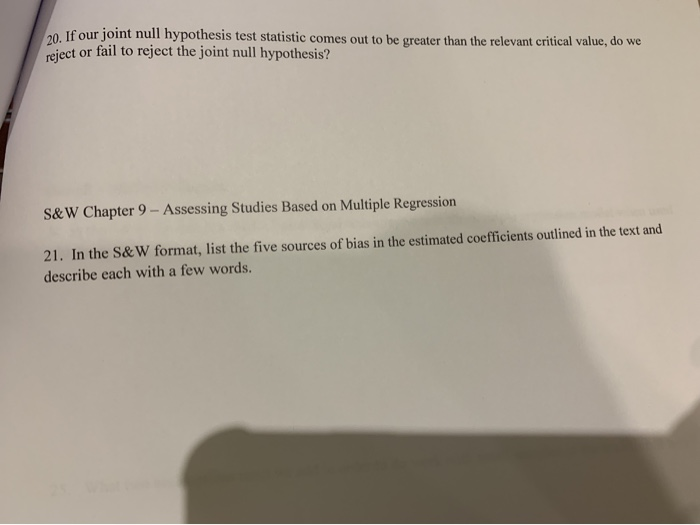r joint null hypothesis test statistic comes . If ou out to be greater than the relevant critical value, do we reject or fail to reject the joint null hypothesis? S&W Chapter 9 -Assessing Studies Based on Multiple Regression 21. In the S&W format, list the five sources of bias in the estimated coefficients outlined in the text and describe each with a few words. r joint null hypothesis test statistic comes . If ou out to be greater than...

• ### A random sample from normal population yielded sample mean=40.8 and sample standard deviation= 6.1, n =...

A random sample from normal population yielded sample mean=40.8 and sample standard deviation= 6.1, n = 15. H0: μ = 32.6, Ha: μ ≠ 32.6, α = 0.05. Perform the hypothesis test and draw your conclusion. Question 3 options: Test statistic: t = 5.21. P-value=0.00013. Reject H0. There is sufficient evidence to support the claim that the mean is different from 32.6. Test statistic: t = 5.21. P-value=1.9E-7 (0.00000019). Do not reject H0. There is not sufficient evidence to support...

• ### test stat crit value- reject or not and p value- rejecr or no 6 of 10...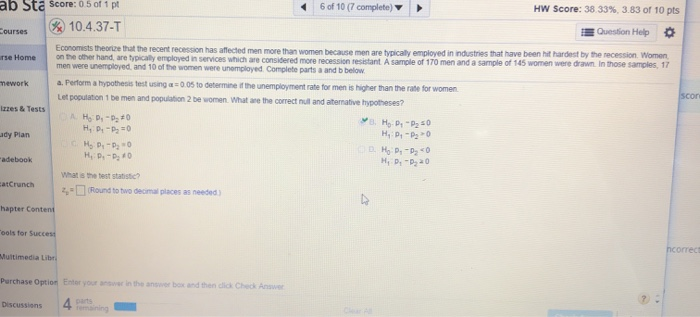test stat crit value- reject or not and p value- rejecr or no 6 of 10 (7 complete) HW Score: 38 33%, 3.83 of 10 pts ab Sta Score: 0.5 of 1 pt 10.4.37-T Courses Question Help urse Home Economists there that the recent recession has affected men more than women because mon are typically employed in industries that have been hit hardest by the recession Women on the other hand, are typically employed in services which are considered more...

• ### b) Identify the P-value and state the researcher’s conclusion if the level of significance was a...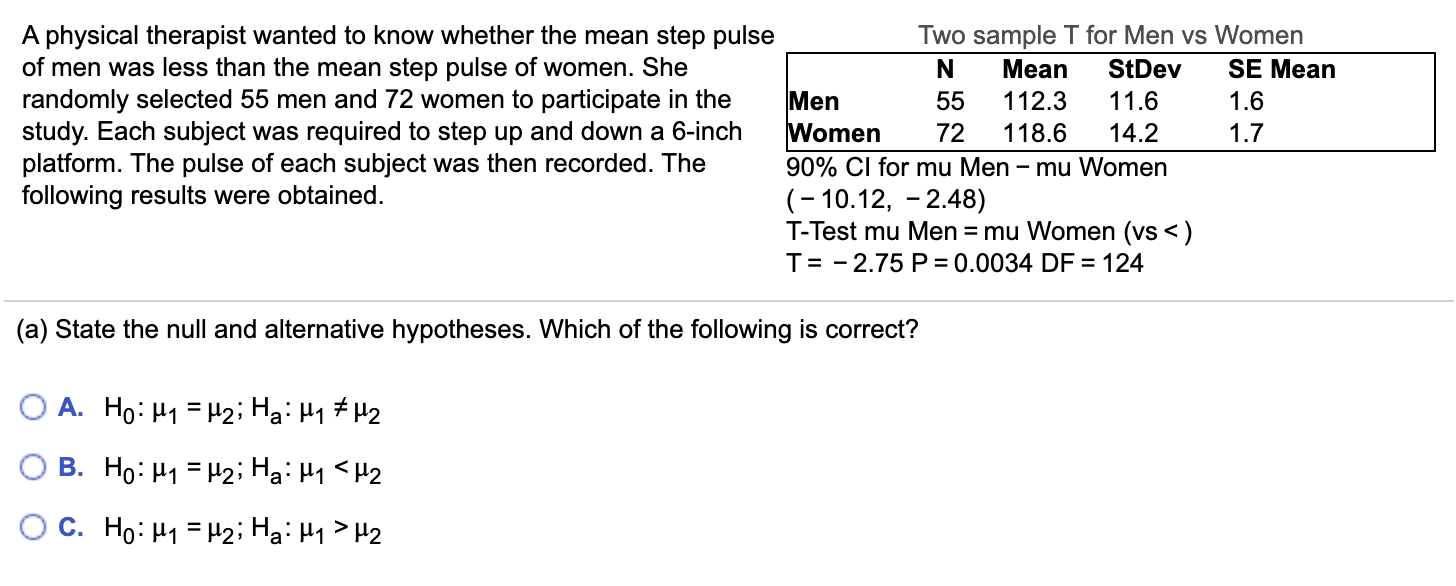b) Identify the P-value and state the researcher’s conclusion if the level of significance was a = 0.001. What is the P-value? P = __ State the researcher’s conclusion. Which of the following is correct? A. Fail to reject H0, there is not sufficient evidence to conclude that the mean step pulse of men was less than the mean step pulse of women. B. Reject H0, there is not sufficient evidence to conclude that the mean step pulse of men...

• ### 5. A study was done on body temperatures of men and women. The results are shown...

5. A study was done on body temperatures of men and women. The results are shown in the table. Assume that the two samples are independent simple random samples selected from normally distributed​ populations, and do not assume that the population standard deviations are equal. Complete parts​ (a) and​ (b) below. Use a 0.01 significance level for both parts. Men Women µ µ1 µ2 N 11 59 xˉ 97.52°F 97.37°F S 0.85°F 0.71°F a. Test the claim that men have...

• ### Test the given claim. Identify the null hypothesis, alternative hypothesis, test statistic, P-value, and then state...

Test the given claim. Identify the null hypothesis, alternative hypothesis, test statistic, P-value, and then state the conclusion about the null hypothesis, as well as the final conclusion that addresses the original claim. Among 2142 passenger cars in a particular region, 249 had only rear license plates. Among 332 commercial trucks, 49 had only rear license plates. A reasonable hypothesis is that commercial trucks owners violate laws requiring front license plates at a higher rate than owners of passenger cars....